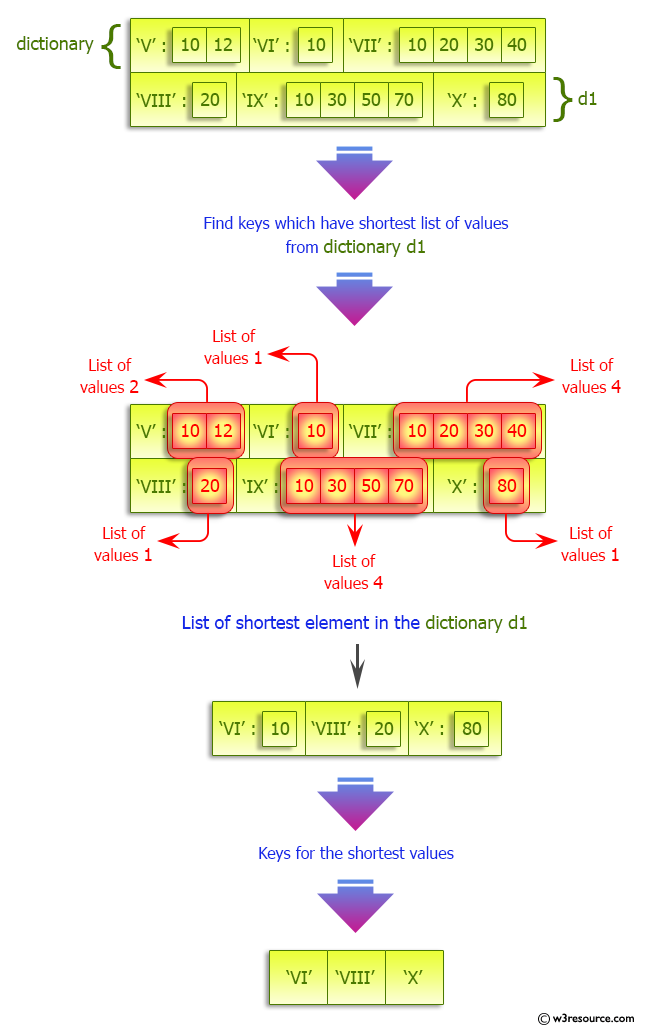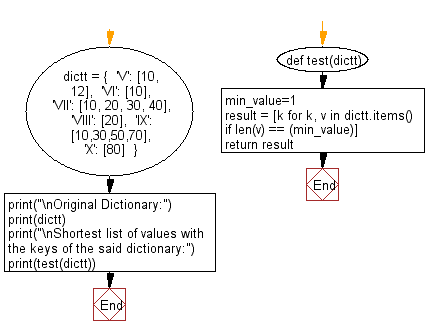﻿ Python: Find shortest list of values with the keys of a dictionary - w3resource# Python: Find shortest list of values with the keys of a dictionary

## Python dictionary: Exercise-60 with Solution

Write a Python program to find shortest list of values with the keys in a given dictionary.

Sample Solution:

Python Code:

``````def test(dictt):
min_value=1
result = [k for k, v in dictt.items() if len(v) == (min_value)]
return result

dictt = {
'V': [10, 12],
'VI': ,
'VII': [10, 20, 30, 40],
'VIII': ,
'IX': [10,30,50,70],
'X': 
}

print("\nOriginal Dictionary:")
print(dictt)
print("\nShortest list of values with the keys of the said dictionary:")
print(test(dictt))
```
```

Sample Output:

```Original Dictionary:
{'V': [10, 12], 'VI': , 'VII': [10, 20, 30, 40], 'VIII': , 'IX': [10, 30, 50, 70], 'X': }

Shortest list of values with the keys of the said dictionary:
['VI', 'VIII', 'X']
```

Pictorial Presentation:Flowchart:## Visualize Python code execution:

The following tool visualize what the computer is doing step-by-step as it executes the said program:

Python Code Editor:

Have another way to solve this solution? Contribute your code (and comments) through Disqus.

What is the difficulty level of this exercise?

Test your Programming skills with w3resource's quiz.

﻿

## Python: Tips of the Day

Invokes the provided function after ms milliseconds:

Example:

```from time import sleep

def tips_delay(fn, ms, *args):
sleep(ms / 1000)
return fn(*args)
print(tips_delay(
lambda x: print(x),
1000,
'w3r'
))
```

Output:

```w3r
None
```